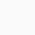# Blog

## Parasitic Computing

By Author – Samata Shelare

It is a generalized problem format into which more specific tasks can be mapped, this opens up the possibility of using the communication protocols provided by Internet hosts as a massive distributed computer. Whats more, the computers that participate in the endeavor are unwitting participants?from their perspective, they are merely responding (or not) to TCP traffic. Parasitic computing is especially interesting because the exploit doesnt compromise the security of the affected computers, it piggybacks a math problem onto the TCP checksum work which TCP enabled hosts to carry out under routine operating conditions.
Parasitic Computing Described:
TCP checksums are normally used to ensure data corruption hasnt occurred somewhere along a packets journey from one computer to another along what is usually a multi-hop route across the Internet. The transmitting computer adds a two-byte checksum field to the TCP header which is a function of the routing information and the data payload of the packet. The idea is that if corruption occurs in the transport or physical layers, the receiving computer will detect this because the presented checksum no longer corresponds with the data received.
Parasitic computing on TCP checksums maps a new problem set onto the TCP checksum function. In the particular instance discussed in BFJB, the technique is to compute a checksum which corresponds to an answer set for a particular boolean satisfiability problem and then to send out packets with data payloads which are potential solutions to that problem. Receiving computers will attempt to validate the checksum against the data payload, effectively checking the solution they were offered the problem under consideration. If the checksum validates, properly configured hosts will reply, and the parasitic computer knows that a solution to the problem has been found. The value of this model is that problems for which there is no known efficient algorithm can be solved by brute-force through parallelization to many hosts.
Schematic illustration of Parasitic Computing from BFJB. Our experiment implemented a modified version of the Parasitic Computing idea: we didnt rely on the HTTP layer as shown in the drawing. We modified the SYN request packet and listened for an SYN-ACK response. Using the handshake step avoids the overhead of establishing the connection beforehand, but may have introduced the false positives we discuss below.
The TCP checksum function breaks the information it checks into 16-bit words and then sums them. Whenever the sum gets larger than 0xffff, the additional column is carried back around (so 0xffff + 0x0001 = 0x0001). The 1s complement of this sum is written down as the checksum. The trick presented in BFJB is to take advantage of the correlation between a numeric sum and a boolean operation: if a and b are bits, and summing results in 2, then upon treatment as booleans a b is TRUE; similarly, if summing a + band results in a 1, then a ? b is TRUE. BFJB provides the truth table showing the relationship between these two boolean operators and the mathematical sum. This is the basis of mapping boolean satisfiability into the TCP checksum space.
In more detail, a boolean satisfiability problem asks whether an assignment of truth-values exists which will allow a given formula to evaluate to TRUE. Typically, these formulae are presented in conjunctive normal form (an AND of ORs). However, the problem exemplified in BFJB allows either ? or to appear in a clause (aside: its noteworthy that is derivable from ? and : [a b] = [a ? b] ? [a b], but more on this below). The example in BFJB is built from the specific case where there are 16 unique variables and 8 clauses.
The first step, then, in finding a solution to this problem parasitically is to calculate a checksum which is in correspondence with an assignment which solves the problem. To do this, BFJB takes each of the 8 clauses in some order and generate a checksum based on the operator involved: for every , write down 10, and for every ?, we write down 01. Then, a checksum is created by taking the 1s complement. This value is the magic checksum which corresponds to a solution to our problem.

After that packets with variable assignments as data payloads can be generated and sent to TCP enabled hosts on the Internet. Each variable is padded with a 0 and then column-aligned with its clause operator according to the ordering used for the checksum creation. The padding is crucial because it is what allows a pair of variables with the operator to sum to 10, without overflowing into the column to the left.
For example, under the assignment where x1 = 1 and x2 = 1 and x3 = 1 and x4 = 0 and x15 = 0 and x16 = 1 we generate two 16 bit words with padding and the operands lined up in columns.
0101…00
0100…01
This packet is then sent to a network host which will evaluate the checksum against the data. Because TCP_CHECKSUM(data) = magic checksum only when the data solves our problem, only those hosts which received a good solution will reply. Each logically possible assignment is generated as a packet payload and sent to a TCP enabled host for evaluation and in this way we have the Internet as a parallel distributed computing for finding solutions to our boolean formulae!
Unfortunately, this relatively high-level description leaves a fair bit of implementation detail to the reader and so we set out to fill in the gaps in order to reproduce the reported results.

Request a Free Estimate
Enter Your Information below and we will get back to you with an estimate within few hours
0